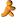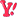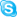Wrox Programmer Forums > .NETcount no of record
 |
 ADO.NET For discussion about ADO.NET.Â Â Topics such as question regarding the System.Data namespace are appropriate.Â Â Questions specific to a particular application should be posted in a forum specific to the application .
 Welcome to the p2p.wrox.com Forums. You are currently viewing the ADO.NET section of the Wrox Programmer to Programmer discussions. This is a community of software programmers and website developers including Wrox book authors and readers. New member registration was closed in 2019. New posts were shut off and the site was archived into this static format as of October 1, 2020. If you require technical support for a Wrox book please contact http://hub.wiley.com

 sanjeev jhaAuthorized User Join Date: Nov 2007 Posts: 16 Thanks: 0 Thanked 0 Times in 0 Postscount no of record

hello
i have two problems firstis i want to insert a value in table when submit at any post always 0 and second when any post is submitin another table then increament it by one there are two table topmaster and reply the column contain for count reply is noofreply i wants to when new post is posted insert 0 value in noofreply in topmaster and when reply posted in reply tabel on that topicid then increament it so please help me
my code for posting is:-(asp)
conn.Open "DSN=ORACLE; User ID = STARTER; Password = STARTER"
If R.Recordcount > 0 Then
R.Movelast
vtopid = R("top_id")
vtopid = CInt(vtopid) + 1
Else
vtopid = 1
End if
R.Close
if R.recordcount>0 then
R.movelast
vopenflg=R("open_flg")
vopenflg=1
end if
r.close
if recordcount>0 then
R.movelast
end if
R.close
If Not IsEmpty(Request.Form("submit")) then
vtopstatus = Request.Form("vtopstatus")
vtopsub = Request.Form("vtopsub")
vopenflg=request.form("vopenflg")
vmsg = Request.Form("vmsg")
vtopsub = trim(vtopsub)
vopenflg=trim(vopenflg)
vmsg = trim(vmsg)
vtopstatus = trim(vtopstatus)
If len(errorMsg) = 0 Then
If len(vtopsub) = 0 Then
errorMsg = "Enter Topic Subject Plese"
End If
End if
If len(errorMsg) = 0 Then
If len(vmsg) = 0 Then
Elseif len(vmsg) > 500 Then
errorMsg = "The voice you are entered is not longer than 500 characters. Please reduce the size."
End If
End If
If len(errorMsg) = 0 Then
conn.Close
conn.Open
R("top_id") = vtopid
R("top_sub") = vtopsub
R("topic_status") = vtopstatus
R("launch_dt") = Cdate(date())
R("open_flg")= vopenflg
R("msg") = vmsg
R.UPdate
R.Close
Response.Redirect "newtopic.asp"
End If
END IF
End If

code for increament if reply is post
code:(asp)
<%
If R.Recordcount > 0 Then
R.Movelast
vrepid = R("rep_id")
vrepid = CInt(vrepid) + 1
Else
vrepid = 1
End if
R.Close
If Not IsEmpty(Request.Form("submit")) then
vtopid=request.form("vtopid")
vtopid=trim(vtopid)
vrepid = trim(vrepid)
If len(errorMsg) = 0 Then
errorMsg = "The voice you are entered is not longer than 500 characters. Please reduce the size."
End If
End If
If len(errorMsg) = 0 Then
conn.Close
conn.Open
R("top_id") =vtopid
R("rep_id") = vrepid
R("rep_dt") = Cdate(date())
R.UPdate
R.Close
conn.close
conn.open
else
End if
end if
R.Update
R.close
If len(errorMsg) > 0 Then
Response.Write "<p><b>" & errorMsg & "</b></p>"
end if
end if
%>

sanjeev
__________________
sanjeev

 melvikFriend of Wrox Join Date: Jun 2003 Posts: 996 Thanks: 2 Thanked 11 Times in 11 PostsDear sanjeev jha:
1_ I didnt get ur problem, sorry.
but anyway I'll do my best if u explain it a bit clearly:)

Always:),
Hovik Melkomian.Similar Threads Thread Thread Starter Forum Replies Last Post record count help jpaul VB Databases Basics 2 January 22nd, 2008 02:49 PM Record count deb1980 ASP.NET 2.0 Basics 6 April 10th, 2007 12:42 PM Count Record prasanta2expert Access 3 November 28th, 2006 10:42 AM How to count a record when it is not there Mitch Access 1 October 3rd, 2003 03:49 PM